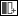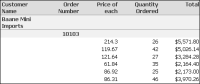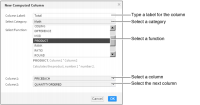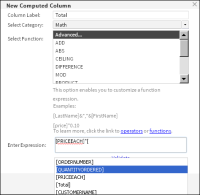Inserting a computed columnTo display calculated data in a report, you create a computed column, such as the Total column in the report shown in Figure 7‑8. In the example, you need to calculate the Total because the original report does not provide this data.Figure 7‑8 Report with computed column
An expression is a statement that produces a value. An expression can be a literal value, such as:
1.23
"Hello, World!"
An expression can contain any combination of literal values, operators, functions, and references to data fields, as shown in the examples in the following sections. In BIRT iHub, Interactive Viewer provides a convenient expression builder wizard to create computed data. You use the expression builder to do one of the following:Enable Interactive Viewer to build an expression.
Select a function to use, then select one or more columns across which Interactive Viewer performs the calculation.Create a custom expression.
Select a function, then create an expression and validate it, after which Interactive Viewer performs the calculation.
Building an expression
The expression builder in Interactive Viewer supports typical mathematical functions, such as percent of total, running sum, and percent of difference. It also supports a range of financial, logical, date‑and‑time, text, and comparison functions.
Figure 7‑9 shows an example of building an expression to calculate the extended price, which is not included in the report.Figure 7‑9 Building an expression
The expression builder contains categories with functions and operators that you use to create and edit expressions. When you build an expression to create a computed column, first select a category, then select an associated function to compute the data. Next, select the columns on which to perform the calculation.
How to build an expression for a new computed column
1 Select and right‑click the column to the left of the location of the new computed column. Choose ColumnNew Computed Column. New Computed Column appears.
2 In Column Label, type a name for the new computed column. The name you specify appears in the column header.
3 In Select Category, select an option from the following categories:FinancialMathDate and timeLogicalComparisonText
A list of functions appears in Select Function.
4 In Select Function, do one of the following:Select a function from the list, then go to step 5.Select Advanced to manually create an expression. Then perform the steps listed in How to create a custom expression for a new computed column.
5 Based on the function you select, one or more column fields appears. In each column field that appears, select a column from the list.
Choose OK. The new computed column, based on the expression you built, appears in the report.
Creating a custom expression
If you are familiar with writing expressions, you can create custom expressions to insert computed columns in a report. In the example shown in Figure 7‑10, you multiply each value in the QUANTITYORDERED field with the corresponding value in the PRICEEACH field, to obtain the value in the Total column. When you use a data field in an expression, you must enclose the field name within brackets ([ ]).
The following section describes examples of custom expressions.Figure 7‑10 Performing a calculation
The following expression displays a customer’s first and last names, which the database stores in two fields. The & operator concatenates string values.
[FirstName] & " " & [LastName]
The following expression displays a full address by concatenating values from four data fields and adding commas as appropriate:
[Address1] & ", " & [City] & ", " & [State] & " " & [Zipcode]
The following expression calculates a gain or loss percentage. The expression uses the mathematical subtraction, division, and multiplication operators, ‑, /, and *.
([SalePrice] ‑ [UnitPrice])/[UnitPrice] * 100
The following expression uses the DIFF_DAY function to calculate the number of days it took to process an order for shipping:
DIFF_DAY([OrderDate], [ShippedDate])
The following expression uses the ADD_DAY function to calculate a payment due date when the payment term is net 30:
The following expression uses the IF function to evaluate if the value in the country column is UK. If the condition is true, the function replaces the value with United Kingdom. If the condition is false, the country values are displayed as stored.
IF(([Country]="UK"),"United Kingdom",[Country])
The following example uses the TRIM function to remove leading or trailing blank characters:
TRIM([CustomerName])
How to create a custom expression for a new computed column
This procedure assumes you have provided a column label, selected a category, and selected the advanced function in New Computed Column.
1 In Enter Expression, type the expression that performs the calculation:To use a data field in the expression, type the left bracket ( [ ), then select the required field from the list that appears. The list displays only fields in the report.To use a function, type the first letter of the function, then select the function from the list that appears. The functions indicate the arguments, if any, that you need to specify.
2 After you complete typing the expression, choose Validate. If the expression is syntactically correct, Information appears, informing you that the expression is valid, as shown in Figure 7‑11.Figure 7‑11 Expression valid confirmation message
Choose OK.
3 In New Computed Column, choose OK. The computed column appears in the report.
Using numbers and dates in a custom expression
When you create an expression that contains a literal number, type the number according to the conventions of the US English locale. In other words, use a period (.), not a comma ( , ) as the decimal separator, even if you are working in, for example, the French locale. For example:
Correct: ([Quantity] * [Price]) * 1.5
Incorrect: ([Quantity] * [Price]) * 1,5
Similarly, when you create an expression that contains a literal date, type the date according to the conventions of the US English locale. For example, if you are working in the French locale, type 03/12/2007 to represent March 12, 2007. Do not type 12/03/2007, which is the convention for the French locale. You must enclose literal date values in double quotation marks (" "), as shown in the following expression that calculates the number of days from the order date to Christmas:
DIFF_DAY([OrderDate], "12/25/08")
How to add days to an existing date value
To create a column that displays date values that are greater than the date values in another column, complete the following steps.1 Select and right‑click a column. Choose ColumnNew Computed Column. New Computed Column appears.
2 In Column Label, type a name for the calculated column. For example, type Forecast Shipping Date.
3 In Enter Expression, type the letter A. A list appears, displaying functions that begin with A.
5 For the first argument, type a left bracket ( [ ) and select the date column from the list. For example, select Order Date.
6 For the second argument, type the number of days to add. In this case, type 7.
7 Validate the expression and choose OK. The calculated column appears in the report. For every value in the Order Date column, the calculated column displays a date seven days later than the order date.
How to subtract date values in a calculated column
The following section describes how to display the difference between two date values.1 Select and right‑click a column. Choose ColumnNew Computed Column. New Computed Column appears.
2 In Column Label, type a name for the calculated column. For example, for a calculation that subtracts the date requested from the actual shipping date, type Shipping Delay.
3 In Enter Expression, type the letter d. A list appears, displaying functions that begin with d.
4 Choose DIFF_DAY(date1, date2).
5 For the first argument, type a left bracket ( [ ) and select the first date column from the list. For example, select Date Requested.
6 For the second argument, type a left bracket ( [ ) and select the second date column from the list. For example, select Actual Shipping Date.
7 Validate the expression and choose OK. The calculated column appears in the report, displaying the difference between the two dates.
Using reserved characters in a custom expression
Some characters are reserved for internal use and have a special meaning. For example, Interactive Viewer uses brackets to denote a data field. The following characters are reserved in Interactive Viewer:
[
]
?
' (single quotation mark)
If the name of a data field contains a reserved character, Interactive Viewer encloses the reserved character in single quotation marks ( ' ) when you select the data field for use in an expression, for example '['. If the name of a data field is OBSOLETE?, Interactive Viewer changes it to [OBSOLETE'?'] in the expression. If you type [OBSOLETE?] in the expression, the dialog box displays an error message.
To minimize syntax errors, select the field from the list in the New Computed Column dialog box and allow the software to construct the expression, instead of typing it yourself. The following examples show the appearance of data fields containing reserved characters in the New Computed Column dialog box. The examples show both versions of the names, the changed name and the original name with reserved characters, as follows:
[ORDER'''S STATUS] ‑ ORDER'S STATUS
[PRODUCTCODE'['4-digit']'] ‑ PRODUCTCODE[4‑digit]
[OBSOLETE'?'] ‑ OBSOLETE?